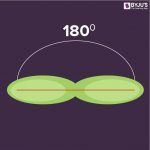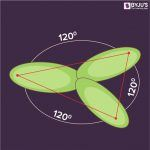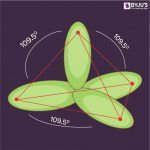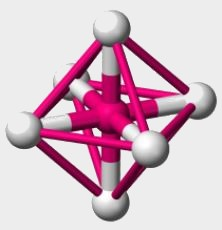# Hybridization

Hybridization in Chemistry is defined as the concept of mixing two atomic orbitals to give rise to a new type of hybridized orbitals. This intermixing usually results in the formation of hybrid orbitals having entirely different energies, shapes, etc. The atomic orbitals of the same energy level mainly take part in hybridization. However, both fully filled and half-filled orbitals can also take part in this process, provided they have equal energy.

On the other hand, we can say that the concept of hybridization is an extension of the valence bond theory and it helps us to understand the formation of bonds, bond energies and bond lengths.

## What is Hybridization?

Redistribution of the energy of orbitals of individual atoms to give orbitals of equivalent energy happens when two atomic orbitals combine to form a hybrid orbital in a molecule. This process is called hybridization.  During the process of hybridization, the atomic orbitals of comparable energies are mixed together and mostly involves the merging of two ‘s’ orbitals or two ‘p’ orbitals or mixing of an ‘s’ orbital with a ‘p’ orbital as well as ‘s’ orbital with a ‘d’ orbital. The new orbitals thus formed are known as hybrid orbitals. More significantly, hybrid orbitals are quite useful in explaining atomic bonding properties and molecular geometry.

Let us quickly look at the example of a carbon atom. This atom forms 4 single bonds wherein the valence-shell s orbital mixes with 3 valence-shell p orbitals. This combination leads to the formation of 4 equivalent sp3 mixtures. These will have a tetrahedral arrangement around the carbon which is bonded to 4 different atoms.

#### Hybridization Video Lesson## Key Features of Hybridization

• Atomic orbitals with equal energies undergo hybridization.
• The number of hybrid orbitals formed is equal to the number of atomic orbitals mixing.
• It is not necessary that all the half-filled orbitals must participate in hybridization. Even completely filled orbitals with slightly different energies can also participate.
• Hybridization happens only during the bond formation and not in an isolated gaseous atom.
• The shape of the molecule can be predicted if the hybridization of the molecule is known.
• The bigger lobe of the hybrid orbital always has a positive sign, while the smaller lobe on the opposite side has a negative sign.

Try This: Give the hybridization states of each of the carbon atoms in the given molecule.

• H2C = CH – CN
• HC ≡ C − C ≡ CH
• H2C = C = C = CH2

## Types of Hybridization

Based on the types of orbitals involved in mixing, the hybridization can be classified as sp3, sp2, sp, sp3d, sp3d2, sp3d3. Let us now discuss the various types of hybridization, along with their examples.

### sp Hybridization

sp hybridization is observed when one s and one p orbital in the same main shell of an atom mix to form two new equivalent orbitals. The new orbitals formed are called sp hybridized orbitals. It forms linear molecules with an angle of 180°

• This type of hybridization involves the mixing of one ‘s’ orbital and one ‘p’ orbital of equal energy to give a new hybrid orbital known as an sp hybridized orbital.
• sp hybridization is also called diagonal hybridization.
• Each sp hybridized orbital has an equal amount of s and p character – 50% s and 50% p character.#### Examples of sp Hybridization:

• All compounds of beryllium like BeF2, BeH2, BeCl2
• All compounds of carbon-containing triple bond like C2H2.

### sp2 Hybridization

sp2 hybridization is observed when one s and two p orbitals of the same shell of an atom mix to form 3 equivalent orbitals. The new orbitals formed are called sp2 hybrid orbitals.

• sp2 hybridization is also called trigonal hybridization.
• It involves mixing of one ‘s’ orbital and two ‘p’ orbital’s of equal energy to give a new hybrid orbital known as sp2.
• A mixture of s and p orbital formed in trigonal symmetry and is maintained at 1200.
• All the three hybrid orbitals remain in one plane and make an angle of 120° with one another. Each of the hybrid orbitals formed has a 33.33% ‘s’ character and 66.66% ‘p’ character.
• The molecules in which the central atom is linked to 3 atoms and is sp2 hybridized have a triangular planar shape.#### Examples of sp2 Hybridization

• All the compounds of Boron i.e. BF3, BH3
• All the compounds of carbon-containing a carbon-carbon double bond, Ethylene (C2H4)

### sp3 Hybridization

When one ‘s’ orbital and 3 ‘p’ orbitals belonging to the same shell of an atom mix together to form four new equivalent orbital, the type of hybridization is called a tetrahedral hybridization or sp3. The new orbitals formed are called sp3 hybrid orbitals.

• These are directed towards the four corners of a regular tetrahedron and make an angle of 109°28’ with one another.
•  The angle between the sp3 hybrid orbitals is 109.280
• Each sp3 hybrid orbital has 25% s character and 75% p character.
• Example of sp3 hybridization: ethane (C2H6), methane.### sp3d Hybridization

sp3d hybridization involves the mixing of 1s orbital, 3p orbitals and 1d orbital to form 5 sp3d hybridized orbitals of equal energy. They have trigonal bipyramidal geometry.

• The mixture of s, p and d orbital forms trigonal bipyramidal symmetry.
• Three hybrid orbitals lie in the horizontal plane inclined at an angle of 120° to each other known as the equatorial orbitals.
• The remaining two orbitals lie in the vertical plane at 90 degrees plane of the equatorial orbitals known as axial orbitals.
• Example: Hybridization in Phosphorus pentachloride (PCl5)### sp3d2 Hybridization

• sp3d2 hybridization has 1s, 3p and 2d orbitals, that undergo intermixing to form 6 identical sp3dhybrid orbitals.
• These 6 orbitals are directed towards the corners of an octahedron.
• They are inclined at an angle of 90 degrees to one another.## Frequently Asked Questions on Hybridization

### What are the different types of hybridization?

Based on the nature of the mixing orbitals, the hybridization can be classified as,

• sp hybridization (beryllium chloride, acetylene)
• sp2 hybridization (boron trichloride, ethylene)
• sp3 hybridization (methane, ethane)
• sp3d hybridization (phosphorus pentachloride)
• sp3d2 hybridization (sulphur hexafluoride)
• sp3d3 hybridization (iodine heptafluoride)

⇒ Know more about VSEPR theory its postulates and limitations

### Among sp, sp2 and sp3, which hybrid orbital is more electronegative?

The percentage of s character in sp, sp2 and sp3 hybridized carbon is 50%, 33.33%, and 25%, respectively.

Due to the spherical shape of the s orbital, it is attracted evenly by the nucleus from all directions. Therefore, a hybrid orbital with more s-character will be closer to the nucleus and thus more electronegative. Hence, the sp hybridized carbon is more electronegative than sp2 and sp3.

### Why is the hybrid orbital during hybridization better than their parent atoms?

The reason why a hybrid orbital is better than their parents:

• Parent s: because it is directional unlike the s orbital.
• Parent p: because it has lower energy than p orbital.

### What are hybrid orbitals?

The hybrid orbitals can be defined as the combination of standard atomic orbitals resulting in the formation of new atomic orbitals.

⇒ Check: Fajan’s Rule and its Postulates

During hybridization, the hybrid orbitals possess different geometry of orbital arrangement and energies than the standard atomic orbitals. Also, the orbital overlap minimizes the energy of the molecule. The degenerate hybrid orbitals formed from the standard atomic orbitals:

• 1s and 1 p: sp orbitals
• 1s and 2p: sp2 orbitals
• 1s and 3p: sp3 orbitals
• 1s, 3p, and 1d: sp3d orbitals
• 1s, 3p, and 2d: sp3d2 orbitals

### What is the difference between sp, sp2 and sp3 hybridization?

sp hybridization occurs due to the mixing of one s and one p atomic orbital, sp2 hybridization is the mixing of one s and two p atomic orbitals and sp3 hybridization is the mixing of one s and three p atomic orbitals.

### What is the percentage of s and p character in sp, sp2 and sp3 hybrid orbital?

The percentage of s and p character in sp, sp2 and sp3 hybrid orbital is,

Sp: s characteristic 50% and p characteristic 50%,

Sp2: s characteristic 33.33% and p characteristic 66.66%,

Sp3: s characteristic 25% and p characteristic 75%.

### Explain the five basic shapes of Hybridization?

The five basic shapes of hybridization are linear, trigonal planar, tetrahedral, trigonal bipyramidal, and octahedral.

The geometry of the orbital arrangement:

• Linear: Two electron groups are involved resulting in sp hybridization, the angle between the orbitals is 180°.
• Trigonal planar: Three electron groups are involved resulting in sp2 hybridization, the angle between the orbitals is 120°.
• Tetrahedral: Four electron groups involved resulting in sp3 hybridization, the angle between the orbitals is 109.5°.
• Trigonal bipyramidal: Five electron groups involved resulting in sp3d hybridization, the angle between the orbitals is 90°, 120°.
• Octahedral: Six electron groups involved resulting in sp3d2 hybridization, the angle between the orbitals is 90°.

### Explain sp3 Hybridization in Methane?

The 2s and all the three (3p) orbitals of carbon hybridize to form four sp3 orbitals. These hybrid orbitals bond with four atoms of hydrogen through sp3-s orbital overlap resulting in CH4 (methane). The geometry of orbital arrangement due to the minimum electron repulsion is tetrahedral.

### Amide molecule looks sp3 hybridized but it is sp2, why?

The general process of hybridization will change if the atom is either enclosed by two or more p orbitals or it has a lone pair to jump into a p orbital. Therefore, in the case of an amide molecule, the lone pair goes into a p orbital to have 3 adjacent parallel p orbitals (conjugation).

### What results in sp, sp2 and sp3 hybridization?

Sp and sp2 hybridization results in two and one unhybridized p orbitals respectively whereas in sp3 hybridization there are no unhybridized p orbitals.

### Explain the difference between molecular and hybrid orbitals.

The interactions between the atomic orbitals of two different atoms result in molecular orbitals, whereas when the atomic orbitals of the same atom interact they form hybrid orbitals.

1. Nityanand Gupta

Very nice

2. c r deshmukh

very useful

3. Thanks for such wonderful knowledge

4. Excellent knowledge thankyou

5. Very useful

6. Very helpful and easy to understand

7. Sayyab khalid

Thanks for such good information

8. Devotha

I have been interested with your presentation

9. Sanyukta

Very useful

10. Manish uikey

Very nice